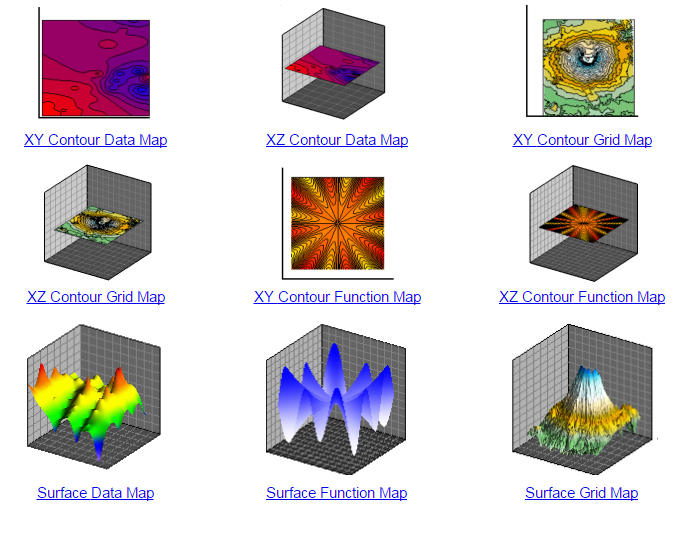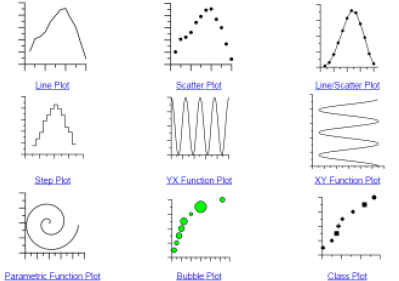# Grapher Plot Types

2D Basic Plots

2D Basic plots are plots that display data as lines, points, and bubbles on two axes. All properties of the plot are editable, including the display of symbols and lines, axes, graph title, and graph background.

Basic plots in Grapher include:

• Line Plots
• Scatter Plots (just points)
• Line & Scatter Plots (lines and points)
• Step Plots
• YX Function Plots
• XY Function Plots
• Parametric Function Plots
• Bubble Plots and Class Plots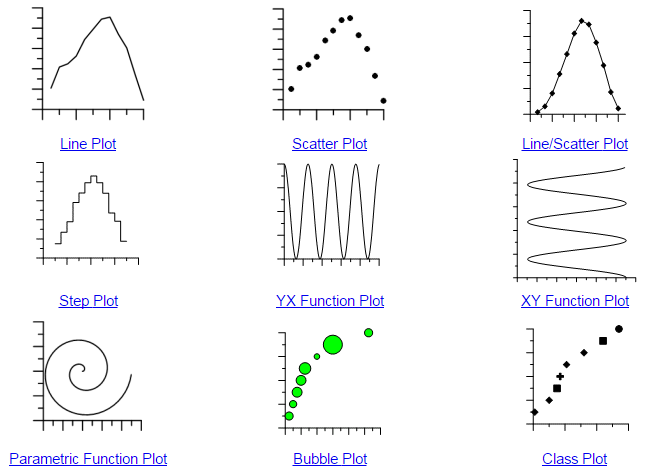3D Basic Plots

3D Basic Plots in Grapher are XY plots extruded to display in 3D.

3D Basic Plots include the following.

• 3D Ribbon Plot
• 3D Wall Plot
• 3D Ribbon Step Plot
• 3D XYZ Plot
• 3D YX Function Plot
• 3D XY Function Plot
• 3D Parametric Function Plot
• 3D Bubble Plot
• 3D Class Plot

Bar Plots

Bar plots display data as bars or floating bars in two and three dimensions. All properties of the plot are editable, including the bar width, bar colors, and number of bars. All properties of the containing graph are also editable, including the axes, graph title, and graph background.

Bar Chart types include:

• Vertical Bar Chart
• Horizontal Bar Chart
• Vertical Floating Bar Chart
• Horizontal Floating Bar Chart
• Vertical Category Bar Chart
• Horizontal Category Bar Chart
• 3D Vertical Bar Chart
• 3D Horizontal Bar Chart
• 3D Vertical Floating Bar Chart
• 3D Horizontal Floating Bar Chart
• 3D XYZ Vertical Bar Chart
• 3D XYZ Horizontal Bar Chart
• 3D XYZ Vertical Floating Bar Chart
• 3D XYZ Horizontal Floating Bar Chart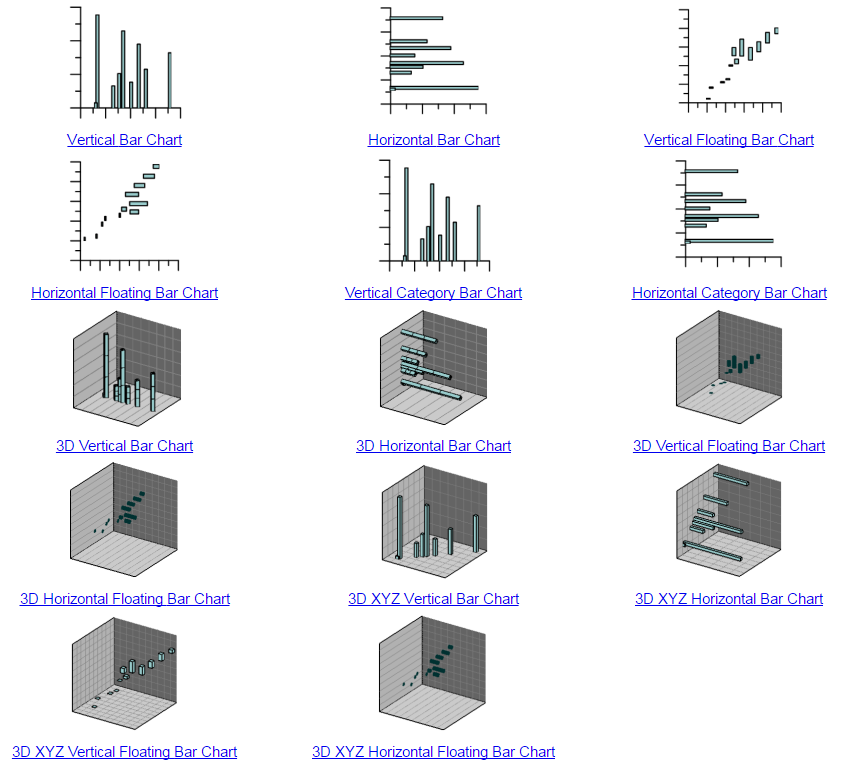Polar Plots

Polar plots display data as lines, points, and bars on polar axes. Data are defined by angle and distance from the center of the plot. Various options exist for each plot type, including setting the axis properties, line properties, symbol properties, and bar properties. Some plots have other properties, such as binning and number of axes.

• Polar Line Plot
• Polar Scatter Plot
• Polar Line/Scatter Plot
• Polar Class Scatter
• 2 Point Polar Vector Plot
• 1 Point Polar Vector Plot
• Function Plot
• Parametric Function Plot
• Polar Bar Chart
• Rose Chart
• Wind Chart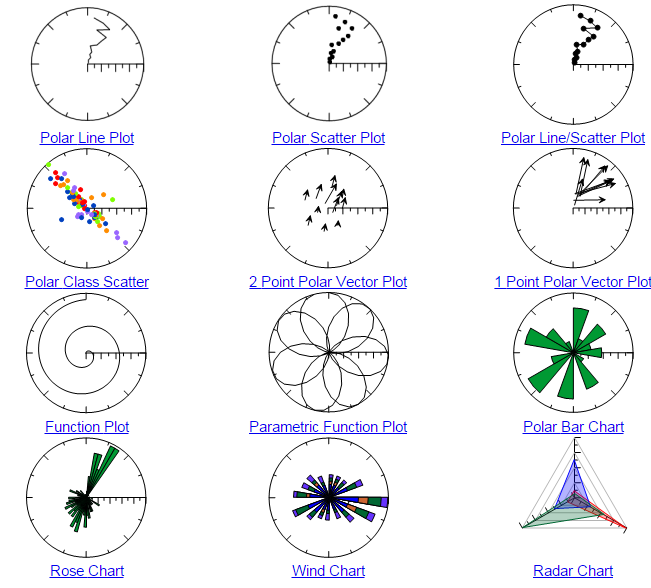Ternary Plots

Ternary plots represent relative percentages in a three component system. Ternary class plots are used to show classification information in the plot. Ternary bubble plots add a fourth dimension to the presented information by varying the symbol size. Various properties, including axis properties, line properties, symbol properties, class properties, and bubble properties can be edited.

Ternary Plot types include:

• Ternary Scatter Plot
• Ternary Line/Scatter Plot
• Ternary Line Plot
• Ternary Class Plot
• Ternary Bubble Plot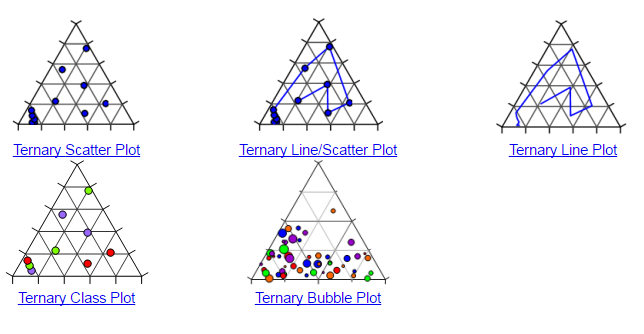Specialty Plots

Specialty plots incorporate many different graph types. Various properties, including axis properties, line properties, bar width properties, number of slices, and method of defining the variables can be edited.

Speciality Plots include the following graph types:

• High-Low-Close Plot
• High-Low-Close Candlestick Plot
• 1 Point Vector Plot
• 2 Point Vector Plot
• 3D 2 Point Vector Plot
• XYZ-dx,dy,dz Vector Plot
• Stiff Plot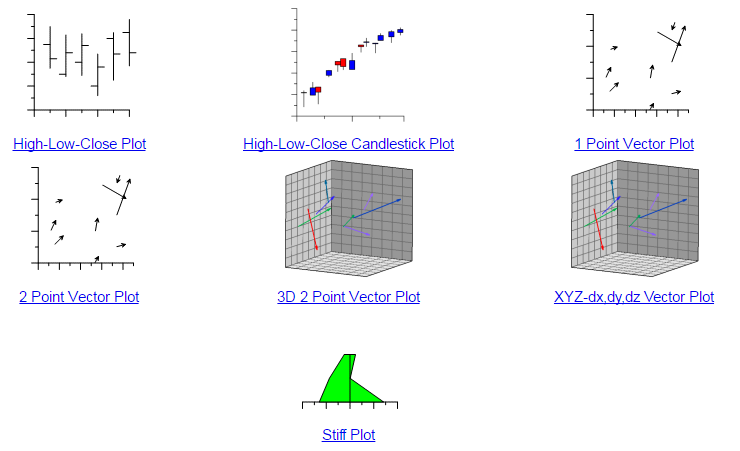Statistical Plots

Statistical plots are plots that display data in a format used to determine some statistical property of the data. Statistical plots can be displayed in two or three dimensions and include a variety of plot types. All properties of the plot are editable, including the display of symbols, lines, and bars. All properties of the containing graph are also editable, including the axes, graph title, and graph background.

Statistical plots include the following types:

• Vertical Histogram
• Horizontal Histogram
• 3D Vertical Histogram
• 3D Horizontal Histogram
• Box-Whisker Plot
• Notched Box-Whisker Plot
• Box-Whisker Plot, Horizontal
• Notched Box-Whisker Plot, Horizontal
• Pie Chart
• 3D XYY Pie Chart
• Q-Q Plot
• Normal Q-Q Plot
• Doughnut Plot
• 3D Doughnut Plot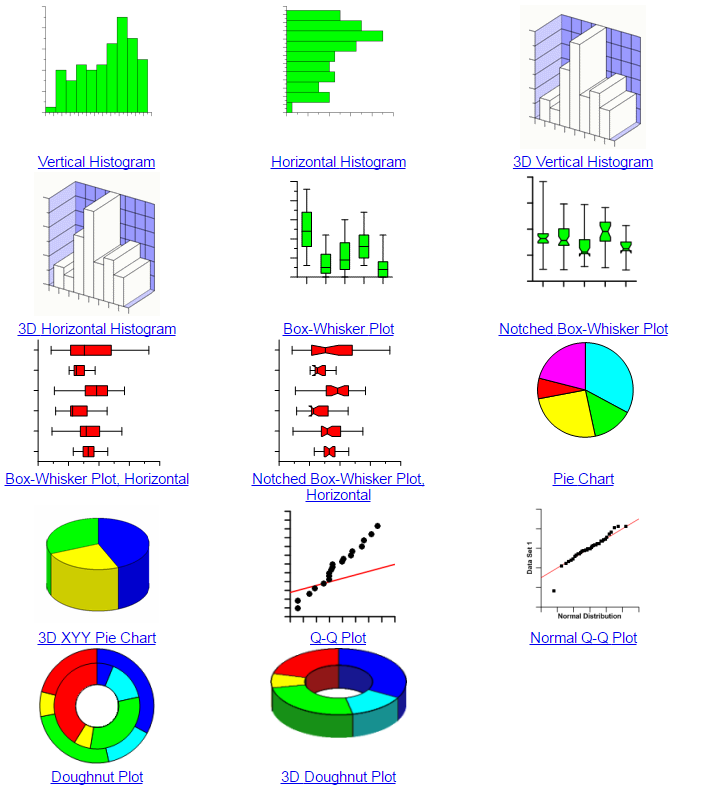Contour Surface Maps

Contour Surface Maps in Grapher display XYZ data as in a similar fashion to mapping software, but the scaling is controlled by the axes lengths instead of setting a particular scale.  Plots are displayed in 2D or 3D.

Contour Surface Maps include:

• XY Contour Data Map
• XZ Contour Data Map
• XY Contour Grid Map
• XZ Contour Grid Map
• XY Contour Function Map
• XZ Contour Function Map
• Surface Data Map
• Surface Function Map
• Surface Grid Map
• 2D Summation